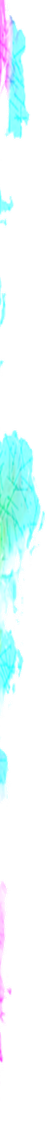## Function Reference

FunctionsUsed
attr()To get selected element's specific attribute value
blur()To blur an element
brightness()To set the brightness of an element
calc()To do some simple calculation
clamp()
circle()To show circular clipping region of an element
contrast()To set the contrast of an element
cubic-bezier()To set the speed curve for animation or transition
ellipse()To show ellipse based clipping region of an element
grayscale()To grayscale an element
hue-rotate()To set the hue-rotate filter on an element
invert()To invert the color an element
inset()To show rectangular clipping region of an element
min()To set the minimum value from specified values to an element
max()To set the maximum value from specified values to an element
opacity()To set the opacity of an element
path()To show path based clipping region of an element
polygon()To show polygon based clipping region of an element
saturate()To saturate an element
sepia()To set the sepia filter on an element
steps()
url()To set the file path you wanna access
var()To access the declared variable

acos()To get trigonometric inverse cos of a number, used in calc( )
asin()To get trigonometric inverse sin of a number, used in calc( )
atan()To get trigonometric inverse tangent of a number, used in calc( )
atan2()To get trigonometric inverse tangent of two numbers, used in calc( )
cos()To get trigonometric cos of a number, used in calc( )
sin()To get trigonometric sin of a number, used in calc( )
tan()To get trigonometric tan of a number, used in calc( )